Welcome To Basics In Maths

# Engineering Mathematics SEM – 1ConceptMathematics Notes for Polytechnic SEM – 1 is Designed by the ” Basics in Maths” team. Here we can learn Concepts in Basic Engineering mathematics Polytechnic Sem – I.

This Material is very Useful for Basic Engineering Mathematics Polytechnic Sem – I Students.

By learning These Notes, Basic Engineering Mathematics Polytechnic Sem – I Students can Write their Exam successfully and fearlessly.

Engineering Mathematics SEM – 1Concept

LOGARITHMS

Logarithm: For ant two positive real numbers a, b, and a ≠ 1. If the real number x such then ax = b, then x is called logarithm of b to the base a. it is denoted by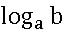####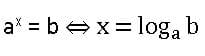Standard formulae of logarithms: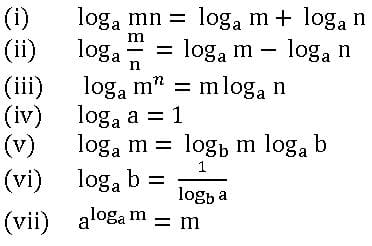Logarithmic Function:

Let a be a positive real number and a ≠ 1. The function f: (o, ∞) → R Defined by f(x) =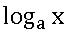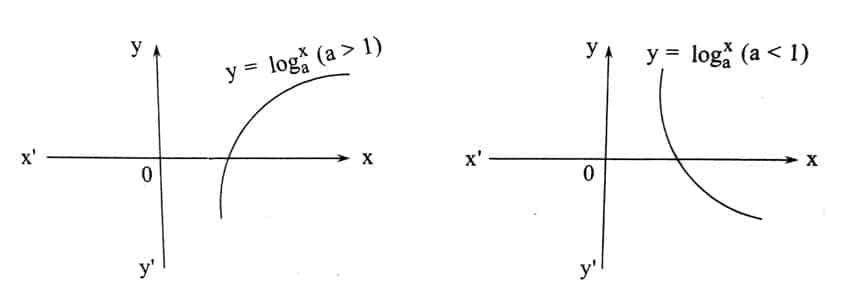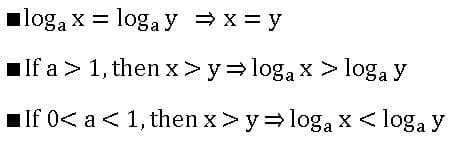PARTIAL FRACTIONS

Fractions:

If f(x) and g(x) are two polynomials, g(x) ≠ 0, then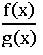is called rational fraction.

Ex:

##### Proper Fraction:

A rational fractionis said to be a Proper fraction if the degree of g(x) is greater than the degree of f(x).

Ex:

Improper Fraction:

A rational fractionis said to be an Improper fraction if the degree of g(x) is less than the degree of f(x).

Ex:

Partial Fractions:

Expressing rational fractions as the sum of two or more simpler fractions is called resolving a given fraction into a partial fraction.

∎ If R(x) =is proper fraction, then

Case(i): – For every factor of g(x) of the form (ax + b) n, there will be a sum of n partial fractions of the form: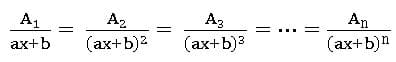Case(ii): – For every factor of g(x) of the form (ax2 + bx + c) n, there will be a sum of n partial fractions of the form: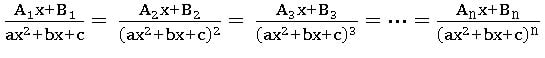∎ If R(x) =is improper fraction, then

Case (i): – If degree f(x) = degree of g(x),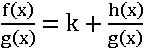where k is the quotient of the highest degree term of f(x) and g(x).

Case (ii): – If f(x) > g(x)